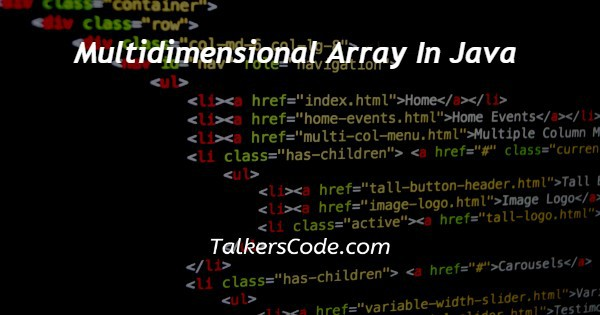# Multidimensional Array In Java

Last Updated : Feb 15, 2023In this article we will show you the solution of multidimensional array in java, a homogenous data structure known as an array holds identical data in clusters of memory.

A multidimensional array can be supposed of as an array within of an array, expressing that the elements within are themselves arrays.

They can store data in tabular form with multiple rows and columns. As far as Java is concerned, two, three, four, five, and more dimensional arrays can be created.

However, managing arrays with more than three tiers is challenging. Now move to the concept of multidimensional array in java.

## Step By Step Guide On Multidimensional Array In Java :-

A collection of values of the same type can be stored in an array as a data structure. The data's integer index can be used to access it.

An array is always initialised with its size, and it cannot be altered while the programme is running as all the data is kept in contiguous memory locations.

Multidimensional arrays, such as a 2D array, are simply a collection of 1D arrays placed together.

A 3D array is similar to many 2D arrays combined into a 1D array, and so forth. Data in multi-dimensional arrays is kept in tabular form, or row-major format.

Multiple indexes are utilised to access array elements in multidimensional arrays.

### Java Multidimensional Array Types

A multidimensional array, where D stands for dimension, can be a 2D array, 3D array, or 4D array.

However, accessing and storing pieces becomes increasingly challenging as the dimension increases.

Many computer games, including simulation games, strategy games, and first-person shooter games, feature characters and objects that exist in two dimensions.

Applications for these positional games depict objects using a two-dimensional array utilising indices, like I and j, which relate to the cells in the array.

A row number is referenced by the first index, while a column number is referenced by the second index. A two-dimensional array in Java is nothing more than a table or matrix with columns and rows.

### A. Indirect Declaration Method

The indirect method entails declaring the array's size up front and filling it with values later.

Either arrayName or DataType can come before the two square brackets ([][]).

Because Java is a statically-typed programming language, the type of element it will take is determined by the DataType parameter.

The variable name assigned to the array that will be used while accessing, modifying, and storing data is arrayName.

### B. Direct Method of Declaration

The array's values are just given upon declaration when using the direct method.

When declaring the two-dimensional array, the two square brackets ([][]) can be used either after DataType or after arrayName.

```class MymultidimensionalArray {
public static void main(String[] args) {
int[][] a = {
{-1, 2, 3},
{4, 5, 6, -9},
{7},
};
for (int[] innerArray: a) {
for(int data: innerArray) {
System.out.println(data);
}
}
}
}```
1. The MymultidimensionalArray class is created in the first line of code.
2. Next, public static void main is used (String[] args).
3. Next, we use an int array to generate a 2D array.
4. Next, we utilise the for loop twice after using it once to access each unique array.
5. We used a second for loop to retrieve each element that was present in a row.
6. Then we print our result with the help of system.out.println.

## Conclusion :-

Hence we have successfully learnt about the concept of muldimensional array in java.

And also we learnt that The same type of data can be stored in arrays, which are homogeneous data structures.

Arrays can have either one or many dimensions.The complexity of multidimensional arrays rises as the number of dimensions grows.

Two, three, and other dimensions are all possible for multidimensional arrays.

A two-dimensional array can be seen as an assortment of one-dimensional arrays.

Similarly, a three-dimensional array can be seen as a collection of two-dimensional arrays.

There are two ways to add elements to the array: directly and indirectly.

They can be accessible in a variety of ways, such as through loops and indexes.

Arrays in two and three dimensions are widely used in graphics, games, software, mathematical analysis, and other fields.

I hope this article on multidimensional array in java helps you and the steps and method mentioned above are easy to follow and implement.